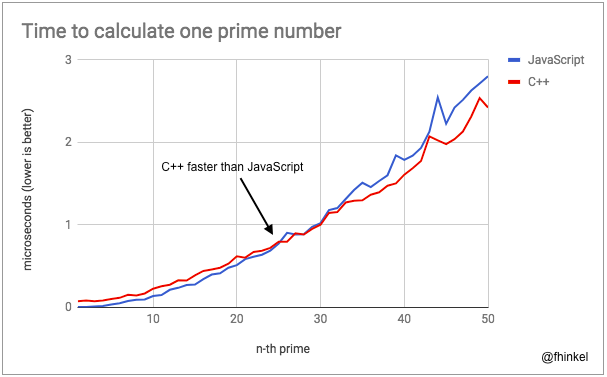Career coaching for women in tech.

# Speed up Your Node.js App with Native Addons

## What’s faster, C++ or JavaScript?

JavaScript is a ridiculously fast scripting language. But how fast is JavaScript compared to C++? Let’s look at this example that computes prime numbers in Node with JavaScript and with a native C++ addon.

The JavaScript and the C++ implementation use the same algorithm. My measurements show that calling into the addon and running the computations in C++ is faster than staying in JavaScript except for small prime numbers.

If your Node app’s sole purpose is to compute prime numbers and you don’t want to use a fast lookup table, please rely on this benchmark 100%. For anything else, this benchmark is probably useless.

Except for the smallest computations, C++ is faster than JavaScript by a factor 1.5, e.g., C++ takes 10 seconds where JavaScript takes 15 seconds. If you have a similar workload (computation heavy, few jumps between C++ and JavaScript), you might want to consider writing a native addon.

V8’s optimizing compiler TurboFan uses information collected at runtime to speed up future computations. We can see in the chart above that TurboFan has finished compiling when we get to the 90th prime number, speeding up JavaScript substantially.Computing the first 50 prime numbers with warm up.

If we “warm up” TurboFan and then start computing prime numbers, JavaScript is faster on the first 25 prime numbers, because calling into C++ comes with a small performance overhead. For larger prime numbers, the C++ addon is faster.

If we use `long` instead of `int` as the parameter for C++’s `prime()` function, it slows down the modulo operator so badly that JavaScript ends up being faster than C++ 🤷🏻‍. Always measure performance, don’t blindly make changes because somebody said “C++ is faster than JavaScript.”

``````const js = require('./primes.js');
const addon = require('./build/Release/primes.node');

function run(i) {
console.time('Prime in js');
js.prime(i);
console.timeEnd('Prime in js');

}

for(let i = 0; i < 1000000; i++) {
run(i);
}
``````

Index.js that runs prime number computations in JavaScript and C++ side by side.

We could easily improve the algorithm. But since we’re not interested in absolute performance, it doesn’t matter as long as both implementations use the same algorithm.

``````function isPrime(p) {
const upper = Math.sqrt(p);
for(let i = 2; i <= upper; i++) {
if (p % i === 0 ) {
return false;
}
}
return true;
}

// Return n-th prime
function prime(n) {
if (n < 1) {
throw Error("n too small: " + n);
}
let count = 0;
let result = 1;
while(count < n) {
result++;
if (isPrime(result)) {
count++;
}
}
return result;
}

module.exports = {
prime
};
``````

N-th prime implementation in JavaScript.

The implementation of the algorithm in `primes.cc` is exactly the same as in `primes.js` modulo syntax. What’s different in the addon is how the function `prime()` is exported:

``````#include <napi.h>
#include <math.h>

namespace primes {

// Implementation of isPrime() and prime() omitted. See

Napi::Value Method(const Napi::CallbackInfo& info) {
Napi::Env env = info.Env();
int number = info.ToNumber().Int32Value();
int res = primes::prime(env, number);
return Napi::Number::New(env, res);
}

Napi::Object Init(Napi::Env env, Napi::Object exports) {
exports.Set(Napi::String::New(env, "prime"),
Napi::Function::New(env, Method));
return exports;
}

NODE_API_MODULE(NODE_GYP_MODULE_NAME, Init)

} // End namespace prime
``````

Initializing the native module with N-API.

This example uses the N-API for the Node.js Native Addon. The N-API is a C API that ensures stability of the Application Binary Interface (ABI) across versions of Node.js. That means, compiled once, the module can be used for different versions of Node (8 and higher) without re-compilation. As C++ is often more convenient than C, we use a C++ wrapper package, node-addon-api. For more info on getting started with N-API check out the documentation or GitHub repository.

`Method` converts the first argument to a number, calls the C++ function `prime` with that number, and returns the result as a JavaScript value. The `Init` function sets `Method` as property `prime` on the exported module.

Except for small prime numbers, C++ is faster than JavaScript. If you have a similar workload (computation heavy, few jumps between C++ and JavaScript) and its performance is critical, you might want to consider writing a native addon.

### Running JavaScript without Optimizations

JavaScript is almost as fast as C++ for tasks like this prime number calculation. Such performance is possible with modern JavaScript engines because they use adaptive optimizations. Without optimizations, the performance difference is much bigger.

In the prime number example, JavaScript without optimizations is 10 times slower than C++, not only 0.5 times. You can turn off optimizations with V8’s `--no-opt` flag, e.g.., by running `node --no-opt index.js`.
The full prime number example is hosted on GitHub. Fork it and experiment with it. Remember to run `npm install` to recompile the addon if you change any C++ code.